# Class 12 RD Sharma Solutions – Chapter 30 Linear Programming – Exercise 30.2 | Set 2

### Question 11. Minimize Z = 5x + 3y

Subject to

2x + y ≥ 10

x + 3y ≥ 15

x ≤ 10

y ≤ 8

x, y ≥ 0

Solution:

Convert the given in equations into equations, we will get the following equations:

2x + y = 10, x + 3y = 15, x = 10, y = 8

Area shown by 2x + y ≥ 10:

The line 2x + y = 10 meets the coordinate axes at A(5, 0) and B(0, 10) respectively.

After connecting these points we will get the line 2x + y = 10.

Thus,

(0,0) does not assure the in equation 2x + y ≥ 10.

Thus,

The area in xy plane which does not have the origin represents the solution set of the in equation 2x + y ≥ 10.

The area represented by x + 3y ≥ 15:

The line x + 3y = 15 connects the coordinate axes at C(15, 0) and D(0, 5) respectively.

After connecting these points we will get the line x + 3y = 15.

Thus,

(0,0) assure the in equation x + 3y ≥ 15.

The area in xy plane which does not have the origin represents the solution set of the in equation x + 3y ≥ 15.

The line x = 10 is the line that passes through the point (10, 0) and is parallel to Y axis.x ≤ 10 is the area to the left of the line

x = 10.

The line y = 8 is the line that passes through the point (0, 8) and is parallel to X axis.y ≤ 8 is the area below the line y = 8.

The area shows by x ≥ 0 and y ≥ 0:

Thus,

All point in the first quadrant assure these in equations.

Thus,

The first quadrant is the area shows by the in equations x ≥ 0 and y ≥ 0.

The suitable area determined by the system of constraints, 2x + y ≥ 10, x + 3y ≥ 15, x ≤ 10, y ≤ 8, x ≥ 0 and y ≥ 0 are as

follows.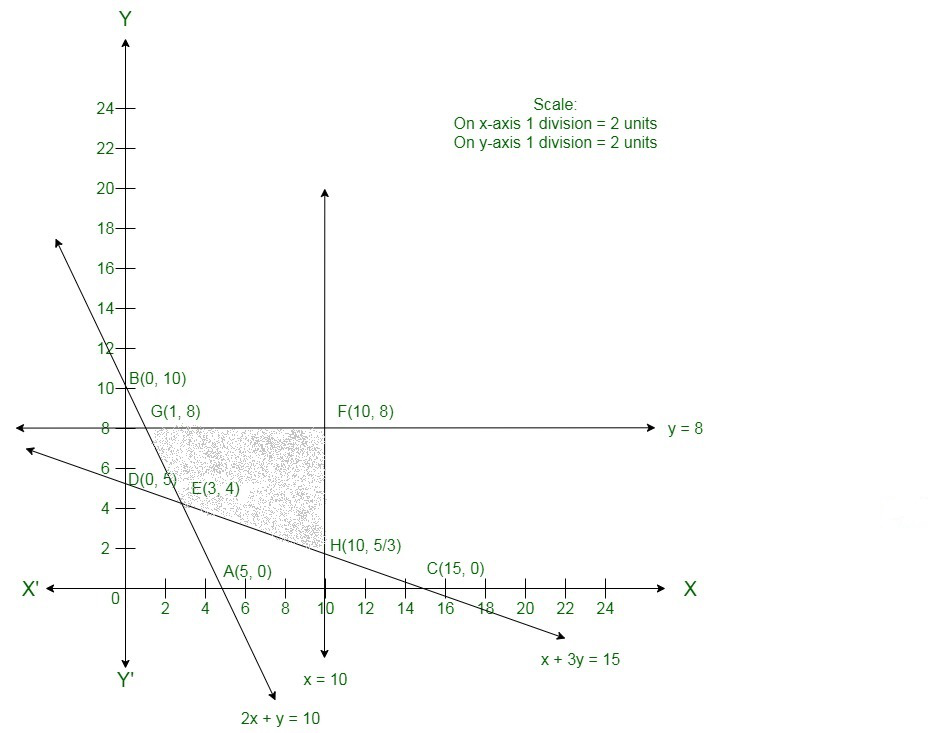The corner points of the suitable region are

E(3, 4),

HF(10, 8) and

G(1, 8).

The values of Z at these corner points are as follows.

Hence,

The minimum value of Z is 27 at the point F(3, 4).

Therefore,

x = 3 and y =4 is the best solution of the given LPP.

Hence, the best value of Z is 27.

### Question 12.  Minimize Z = 30x + 20y

Subject to

x + y ≤ 8

x + 4y ≥ 12

5x + 8y = 20

x, y ≥ 0

Solution:

Convert the given in equations into equations, we will get the following equations:

x + y = 8, x + 4y = 12, x = 0 and y = 0

5x + 8y = 20 is already an equation.

The area shows by x + y ≤ 8:

The line x + y = 8 connects the coordinate axes at A(8, 0) and B(0, 8) respectively.

After connecting these points we will get the line x + y = 8.

Thus,

(0,0) assures the in equation x + y ≤ 8.

Thus,

The area in xy plane which have the origin represents the solution set of the in equation x + y ≤ 8.

The area shows by x + 4y ≥ 12:

The line x + 4y = 12 connects the coordinate axes at C(12, 0) and D(0, 3) respectively.

After connecting these points we will get the line x + 4y = 12.

Thus,

(0,0) assure the in equation x + 4y ≥ 12.

Thus,

The area in xy plane which does not have the origin shows the solution set of the in equation x + 4y ≥ 12.

The line 5x + 8y = 20 is the line that passes through E(4, 0) and F.

The area shows by x ≥ 0 and y ≥ 0:

Thus,

All point in the first quadrant assure these in equations.

Thus,

The first quadrant is the area shows by the in equations x ≥ 0 and y ≥ 0.

The suitable area determined by the system of constraints, x + y ≤ 8, x + 4y ≥ 12, 5x + 8y = 20, x ≥ 0 and y ≥ 0 are as follows.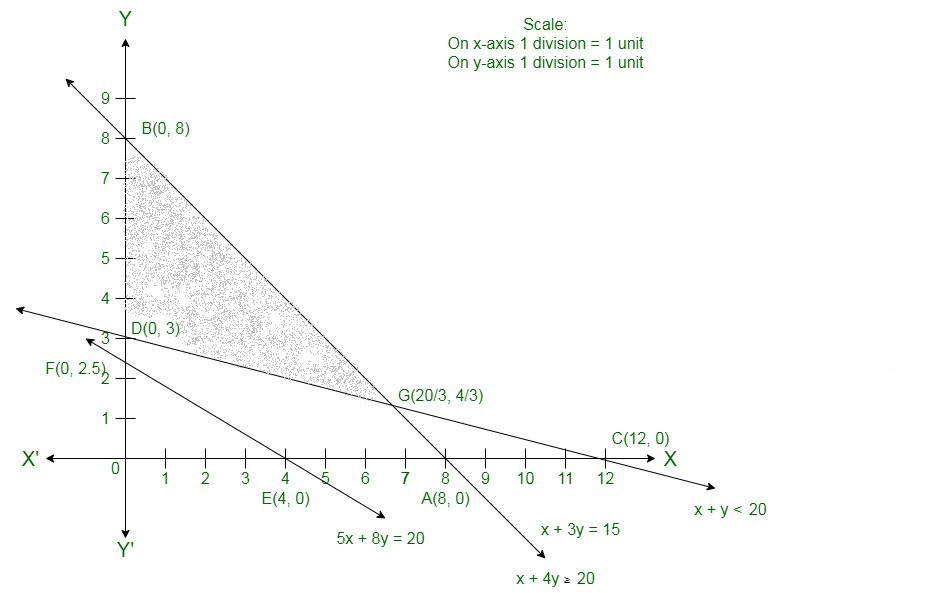The corner points of the suitable area are B(0,8), D(0,3), G.
The values of Z at these corner points are as follows.

Hence,

The minimum value of Z is 60 at the point D(0,3).

Therefore,

x = 0 and y =3 is the best solution of the given LPP.

Therefore,

The best value of Z is 60.

### Question 13.  Maximize Z = 4x + 3y

Subject to

3x + 4y ≤ 24

8x + 6y ≤ 48

x ≤ 6000

x ≤ y

x, y ≥ 0

Solution:

Here, we need to maximize Z = 4x + 3y

Convert the given in equations into equations, we will get the following equations:

3x + 4y = 24, 8x + 6y = 48, x = 5 , y = 6, x = 0 and y = 0.

The line 3x + 4y = 24 connects the coordinate axis at A(8, 0) and B(0,6).

Connects these points to get the line 3x + 4y = 24.

Thus,

(0, 0) assure the in equation 3x + 4y ≤ 24.

Thus,

The area in xy-plane that have the origin represents the solution set of the given equation.

The line 8x + 6y = 48 connects the coordinate axis at C(6, 0) and D(0,8).

Connect these points to get the line 8x + 6y = 48.

Thus,

(0, 0) assure the in equation 8x + 6y  ≤ 48.

Thus,

The area in xy-plane that have the origin represents the solution set of the given equation.

x = 5 is the line passing through x = 5 parallel to the Y axis.

y = 6 is the line passing through y = 6 parallel to the X axis.

The area shown by x ≥ 0 and y ≥ 0:

Hence,

All the points in the first quadrant assure these in equations.

Thus,

The first quadrant is the area shown by the in equations.

These lines are drawn using a suitable scale.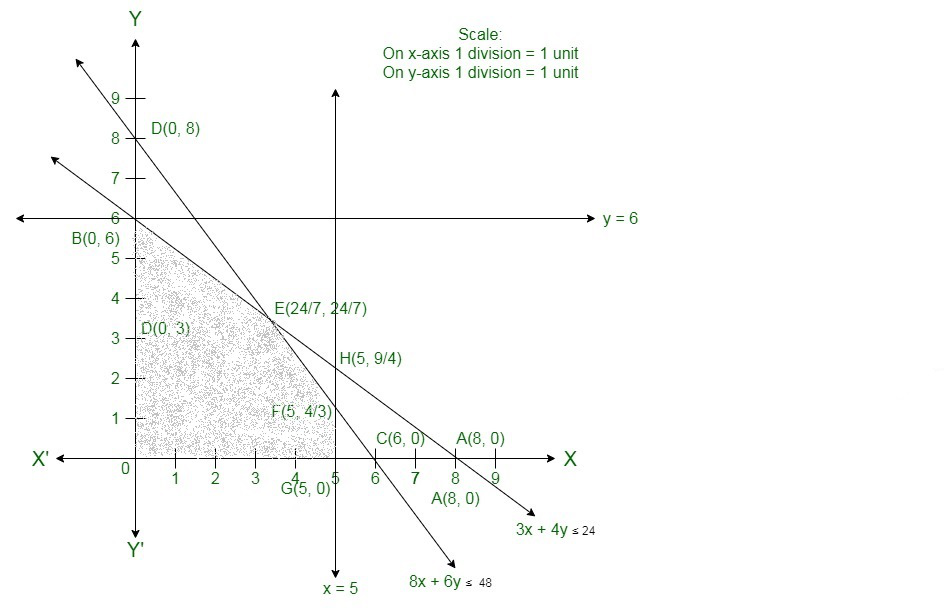The corner points of the feasible region are O(0, 0), G(5, 0), F, Eand B(0, 6).

The values of Z at these corner points are as follows.

Here we can see that the maximum value of the objective function Z is 24 which is at Fand E.

Therefore,

The best value of Z is 24.

### Question 14. Minimize Z = x – 5y + 20

Subject to

x – y ≥ 0

-x + 2y ≥ 2

x ≥ 3

y ≤  4

x, y ≥ 0

Solution:

Convert the given in equations into equations, we will get the following equations:

x − y = 0, − x + 2y = 2, x = 3, y = 4, x = 0 and y = 0.

The area shown by x − y ≥ 0 or x ≥ y:

The line x − y = 0 or x = y passes through the origin.The area to the right of the line x = y will assure the given in equation.

Now we will check by taking an example like if we take a point (4, 3) to the right of the line x = y .

Here, x ≥ y.

Thus,

It assure the given in equation.

Take a point (4, 5) to the left of the line x = y. Here, x ≤ y. That means it does not assure the given in equation.

The area shown by − x + 2y ≥ 2:

The line − x + 2y = 2 connects the coordinate axes at A(−2, 0) and B(0, 1) respectively.

After connecting these points we will get the line − x + 2y = 2.

Thus,

(0,0) does not assure the in equation − x + 2y ≥ 2.

Thus,

The area in xy plane which does not have the origin represents the solution set of the in equation − x + 2y ≥ 2 .

The line x = 3 is the line that passes through the point (3, 0) and is parallel to Y axis. x ≥ 3 is the area to the right of the

line x = 3.

The line y = 4 is the line that passes through the point (0, 4) and is parallel to X axis. y ≤ 4 is the area below the line y = 4.

The area shown by x ≥ 0 and y ≥ 0:

Hence,

All point in the first quadrant assure these in equations.

Thus, the first quadrant is the area shown by the in equations x ≥ 0 and y ≥ 0.

The suitable area determined by the system of constraints x − y ≥ 0,− x + 2y ≥ 2, x ≥ 3, y ≤ 4, x ≥ 0 and y ≥ 0 are as follows.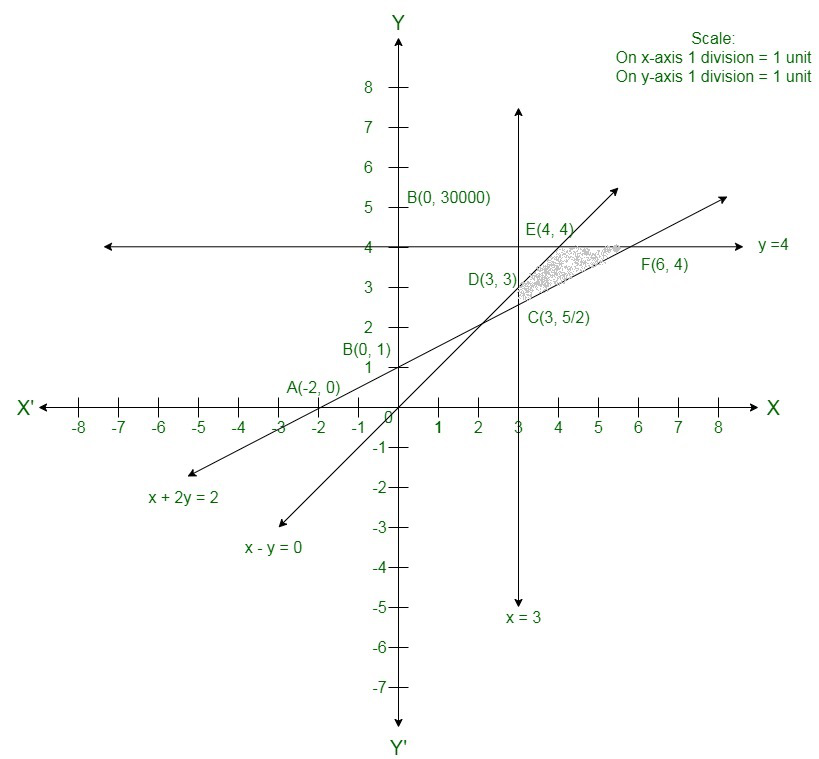The corner points of the suitable area are C, D(3, 3), E(4, 4) and F(6, 4).

The values of Z at these corner points are as follows.

Hence,

The minimum value of Z is 4 at the point E(4, 4).

Therefore,

x = 4 and y = 4 is the best solution of the given LPP.

Hence,

The best value of Z is 4.

### Question 15.  Maximize Z = 3x + 5y

Subject to

x + 2y ≤ 20

x + y ≤ 15

y ≤ 15

x, y ≥ 0

Solution:

Here we need to maximize Z = 3x + 5y

Convert the given in equations into equations, we will get the following equations:

x + 2y = 20, x + y = 15, y = 5, x = 0 and y = 0.

The line x + 2y = 20 connects the coordinate axis at A(20, 0) and B(0,10).

Connect these points to get the line x + 2y = 20.

Thus,

(0, 0) assume the in equation x + 2y ≤ 20.

Thus,

The area in xy-plane that have the origin represents the solution set of the given equation.

The line x + y = 15 connect the coordinate axis at C(15, 0) and D(0,15).

Connect these points to get the line x + y = 15.

Thus,

(0, 0) assume the in equation x + y ≤ 15.

Thus,

The area in xy-plane that have the origin represents the solution set of the given equation.

y = 5 is the line passing through (0, 5) and parallel to the X axis. The area below the line y = 5 will assure the given in equation.

The area shown by x ≥ 0 and y ≥ 0:

Hence,

All the point in the first quadrant assure these in equations.

Thus,

The first quadrant is the area shown by the in equations.

These lines are drawn using a suitable scale.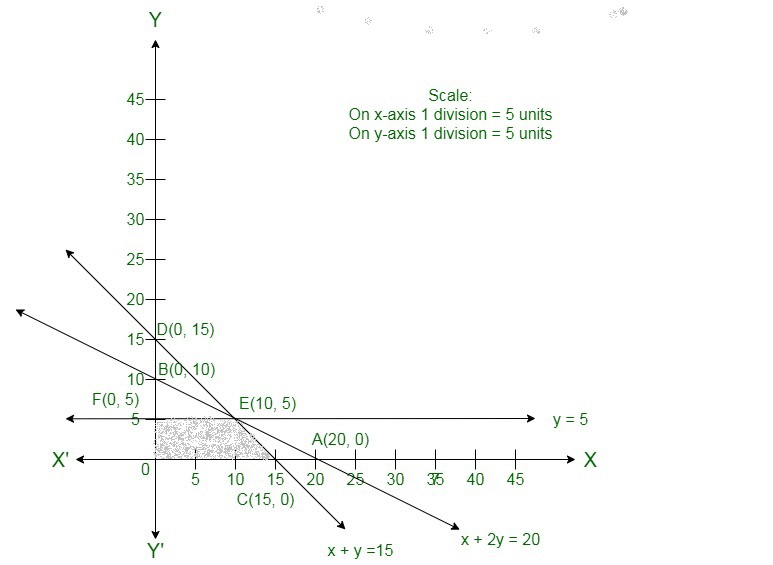The corner points of the suitable area are O(0, 0), C(15, 0), E(10, 5) and F(0, 5)

The values of Z at these corner points are as follows.

Here we can see that the maximum value of the objective function Z is 55 which is at E(10, 5).

Therefore,

The best value of Z is 55.

### Question 16. Minimize Z = 3x1 + 5x2

Subject to

x1 + x2 ≥ 3

x1 + x2 ≥ 2

x1, x2 ≥ 0

Solution:

Convert the given in equations into equations, we will get the following equations:

x1 + 3x2 = 3, x1 + x2 = 2, x1 = 0 and x2 = 0

The area shown by x1 + 3x2 ≥ 3 :

The line x1 + 3x2 = 3 connects the coordinate axes at A(3, 0) and B(0, 1) respectively.

After connecting these points we will get the line x1 + 3x2 = 3.

Thus,

(0,0) does not assure the in equation x1 + 3x2 ≥ 3.

Thus,

The area in the plane which does not have the origin represents the solution set of the in equation x1 + 3x2 ≥ 3.

The area shown by x1 + x2 ≥ 2:

The line x1 + x2 = 2 connects the coordinate axes at C(2, 0) and D(0, 2) respectively.

After connecting these points we will get the line x1 + x2 = 2.

Thus,

(0,0) does not assure the in equation x1 + x2 ≥ 2.

Thus,

The area having the origin represents the solution set of the in equation x1 + x2 ≥ 2.

The area shown by x1 ≥ 0 and x2 ≥ 0:

Hence,

All the points in the first quadrant assure these in equations.

Thus,

The first quadrant is the area shown by the in equations x1 ≥ 0 and x2 ≥ 0.

The suitable area determined by the system of constraints, x1 + 3x2 ≥ 3 , x1 + x2 ≥ 2, x1 ≥ 0, and x2 ≥ 0, are as follows.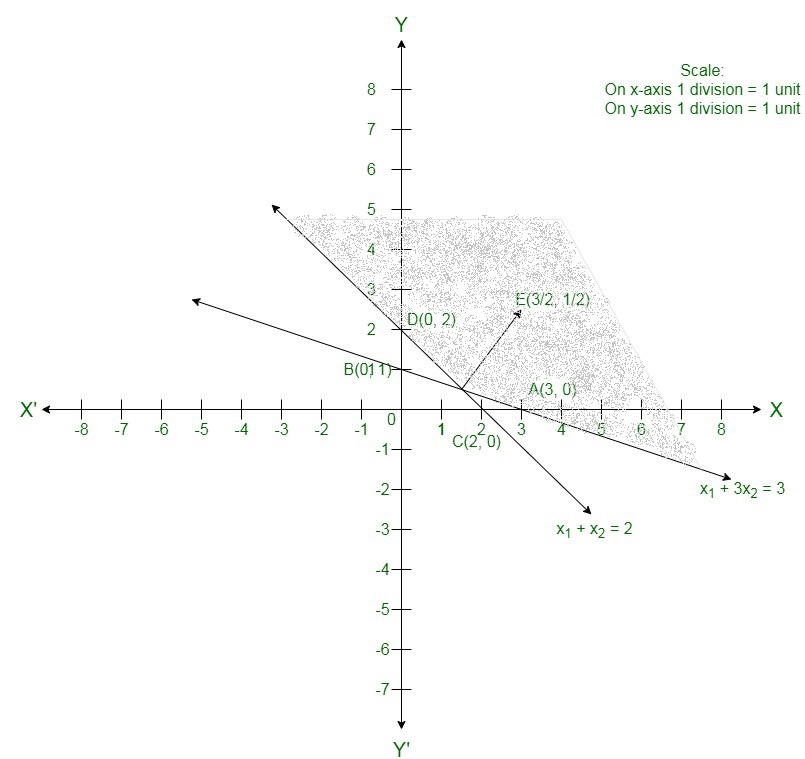The corner points of the suitable area are O(0, 0), B(0, 1), Eand C(2, 0).

The values of Z at these corner points are as follows.

Hence,

The minimum value of Z is 0 at the point O(0, 0).

Hence,

x1 = 0 and x2 = 0 is the best solution of the given LPP.

Therefore,

The best value of Z is 0.

### Question 17.  Maximize Z = 2x + 3y

Subject to

x + y ≥ 1

10x + y ≥ 5

x + 10y ≥ 1

x, y ≥ 0

Solution:

Convert the given in equations into equations, we will get the following equations:

x + y = 1, 10x +y = 5, x + 10y = 1, x = 0 and y = 0

The area shown by x + y ≥ 1:

The line x + y = 1 connects the coordinate axes at A(1, 0) and B(0,1) respectively.

After connecting these points we will get the line x + y = 1.

Thus,

(0,0) does not assure the in equation x + y ≥ 1.

Thus,

The area in xy plane which does not have the origin represents the solution set of the in equation x + y ≥ 1.

The area shown by 10x +y ≥ 5:

The line 10x +y = 5 connect the coordinate axes at Cand D(0, 5) respectively.

After connecting these points we will get the line 10x +y = 5.

Thus,

(0,0) does not assure the in equation 10x +y ≥ 5.

Thus, the area which does not have the origin represents the solution set of the in equation 10x +y ≥ 5.

The area shown by x + 10y ≥ 1:

The line x + 10y = 1 connect the coordinate axes at A(1, 0) and Frespectively.

After connecting these points we will have the line x + 10y = 1.

Thus,

(0,0) does not assure the in equation x + 10y ≥ 1.

Thus, the area which does not have the origin represents the solution set of the in equation x + 10y ≥ 1.

The area shown by x ≥ 0 and y ≥ 0:

Here,

All the points in the first quadrant assure these in equations.

Thus,

The first quadrant is the area shown by the in equations x ≥ 0, and y ≥ 0.

The suitable area determined by the system of constraints x + y ≥ 1, 10x +y ≥ 5, x + 10y ≥ 1, x ≥ 0, and y ≥ 0, are as follows.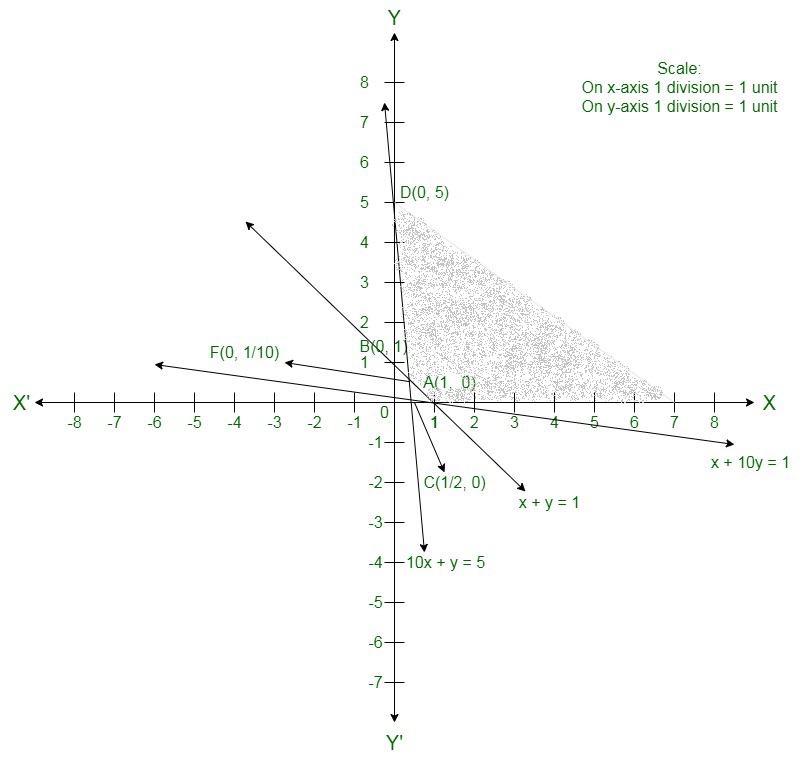The suitable area is unbounded.

Hence,

The maximum value is infinity i.e. the solution is unbounded.

### Question 18. Maximize Z = -x1 + 2x2

Subject to

-x1 + 3x2 ≤ 10

x1 + x2 ≤ 6

x1 – x2 ≤ 2

x1, x2 ≥ 0

Solution:

Convert the given in equations into equations, we will get the following equations:

−x1 + 3x2 = 10, x1 + x2 = 6, x1 + x2 = 2, x1 = 0 and x2 = 0

The area shown by −x1 + 3x2 ≤ 10:

The line −x1 + 3x2 = 10 coincide the coordinate axes at A(−10, 0) and Brespectively.

After connecting these points we will get the line −x1 + 3x2 = 10.

Thus,

(0,0) satisfies the in equation −x1 + 3x2 ≤ 10 .

Thus,

The area region in the plane which have the origin shows the solution set of the in equation

−x1 + 3x2 ≤ 10.

The area shown by x1 + x2 ≤ 6:

The line x1 + x2 = 6 connects the coordinate axes at C(6, 0) and D(0, 6) respectively.

After connecting these points we will get the line x1 + x2 = 6.

Thus,

(0,0) assure the in equation x1 + x2 ≤ 6.

Thus,

The area having the origin represents the solution set of the in equation x1 + x2 ≤ 6.

The area shown by x1− x2 ≤ 2:

The line x1 − x2 = 2 coincide the coordinate axes at E(2, 0) and F(0, −2) respectively.

After connecting these points we will get the line x1 − x2 = 2.

Thus,

(0,0) assure the in equation x1− x2 ≤ 2.

Thus,

The area having the origin represents the solution set of the in equation x1− x2 ≤ 2.

The area shown by x1 ≥ 0 and x2 ≥ 0:

Hence,

All the points in the first quadrant assure these in equations.

Thus,

The first quadrant is the region shown by the in equations x1 ≥ 0 and x2 ≥ 0.

The suitable area determined by the system of constraints, −x1 + 3x2 ≤ 10, x1 + x2 ≤ 6, x1− x2 ≤ 2, x1 ≥ 0, and x2 ≥ 0, are as follows.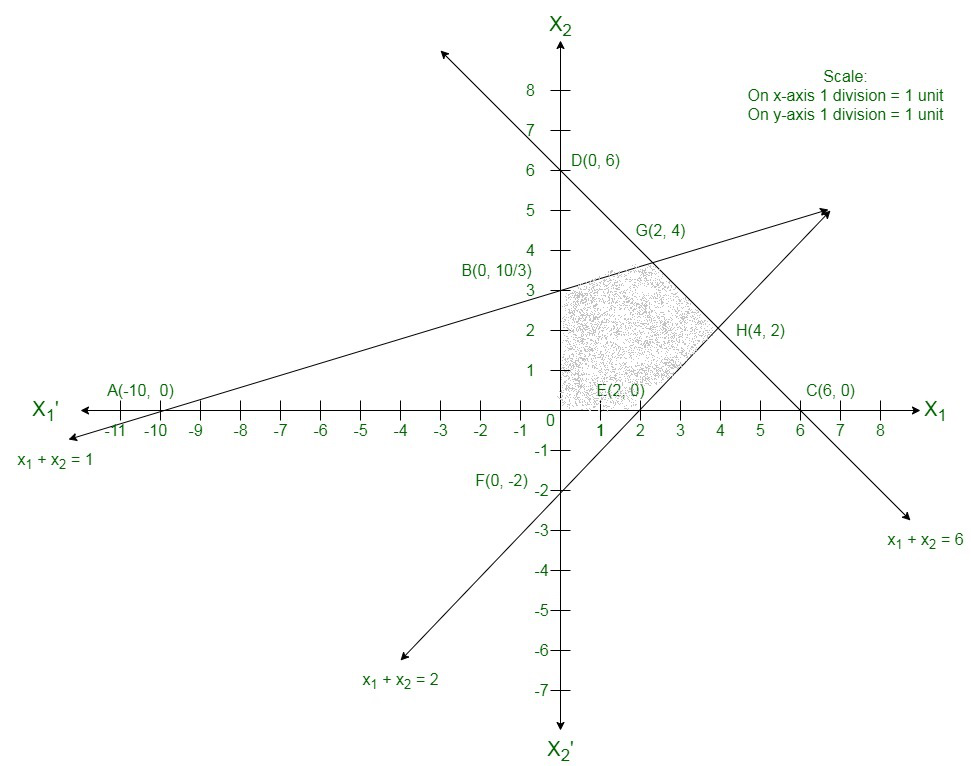The corner points of the assure area are O(0, 0), E(2, 0), H(4, 2), G(2, 4) and B.

The values of Z at these corner points are as follows.

Here we can see that the maximum value of the objective function Z iswhich is at B.

### Question 19. Maximize Z = -x + y

Subject to

-2x + y ≤ 1

x  ≤ 2

x + y ≤ 3

x, x ≥ 0

Solution:

Here we need to maximize Z = x + y

Convert the given in equations into equations, we will get the following equations:

−2x + y = 1, x = 2, x + y = 3, x = 0 and y = 0.

The line −2x + y = 1 coincide the coordinate axis at Aand B(0, 1).

Now connect these points to get the line −2x + y = 1 .

Thus,

(0, 0) assure the in equation −2x + y ≤ 1.

Thus,

The area in xy-plane that have the origin represents the solution set of the given equation.

x = 2 is the line passing through (2, 0) and parallel to the Y axis.

The area below the line x = 2 will assure the given in equation.

The line x + y = 3 coincide the coordinate axis at C(3, 0) and D(0, 3).

Connect these points to get the line x + y = 3.

Thus,

(0, 0) assure the in equation x + y ≤ 3.

Thus,

The area in xy-plane that have the origin shows the solution set of the given equation.

The area shown by x ≥ 0 and y ≥ 0:

Hence,

All the points in the first quadrant assures these in equations.

Thus,

The first quadrant is the area shown by the in equations.

These lines are drawn using a satisfactory scale.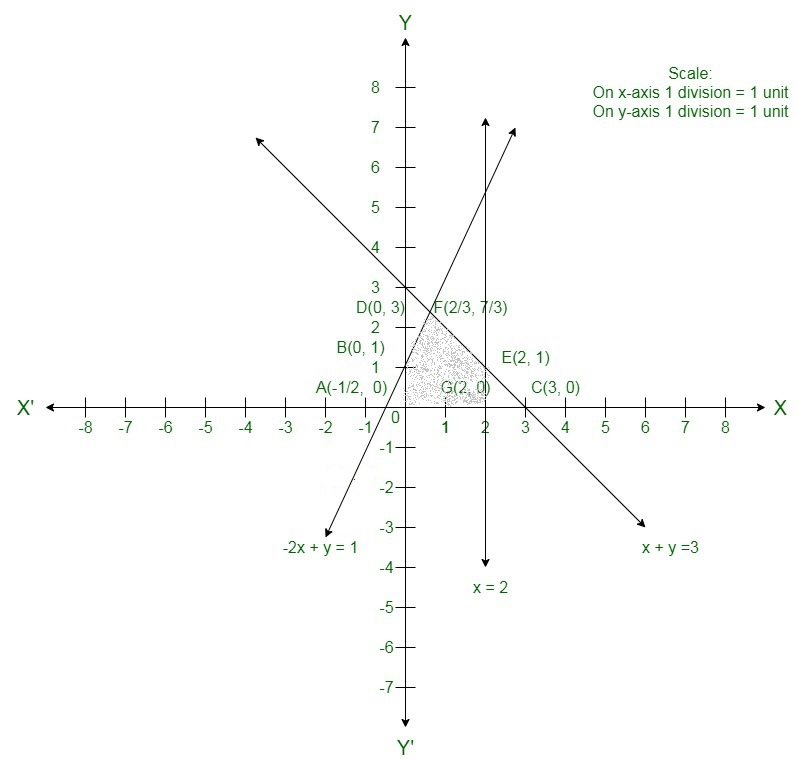The corner points of the suitable area are O(0, 0),G(2, 0), E(2, 1) and FThe values of Z at these corner points are as follows.

Here we can see that the maximum value of the objective function Z is 3 which is at E(2, 1) and F.

Therefore,

The best value of Z is 3.

### Question 20. Maximize Z = -3x1 + 4x2

Subject to

x1 – x2 ≤ -1

x1 + x2  ≤ 0

x1, x2 ≥ 0

Solution:

Convert the given in equations into equations, we will get the following equations:

x1 − x2 = −1, −x1 + x2 = 0, x1 = 0 and x2 = 0

The area shown by x1 − x2 ≤ −1:

The line x1 − x2 = −1 coincide the coordinate axes at A(−1, 0) and B(0, 1) respectively.

After connecting these points we will get the line x1 − x2 = −1.

Thus,

(0,0) does not assures the in equation x1 − x2 ≤ −1 .

Thus,

The area in the plane which does not have the origin shows the solution set of the in equation x1 − x2 ≤ −1.

The area shown by −x1 + x2 ≤ 0 or x1 ≥ x2:

The line −x1 + x2 = 0 or x1 = x2 is the line passing through (0, 0).

The area to the right of the line x1 = x2 will assure the given in equation −x1 + x2 ≤ 0.

If we mark a point (1, 3) to the left of the line x1 = x2

Here, 1≤3 which is not assuring the in equation x1 ≥ x2

Hence,

The area to the right of the line x1 = x2 will assure the given in equation −x1 + x2 ≤ 0.

The area shown by x1 ≥ 0 and x2 ≥ 0:

Thus,

All the points in the first quadrant assure these in equations.

Thus,

The first quadrant is the area shown by the in equations x1 ≥ 0 and x2 ≥ 0.

The suitable area determined by the system of constraints, x1 − x2 ≤ −1, −x1 + x2 ≤ 0, x1 ≥ 0, and x2 ≥ 0, are as follows.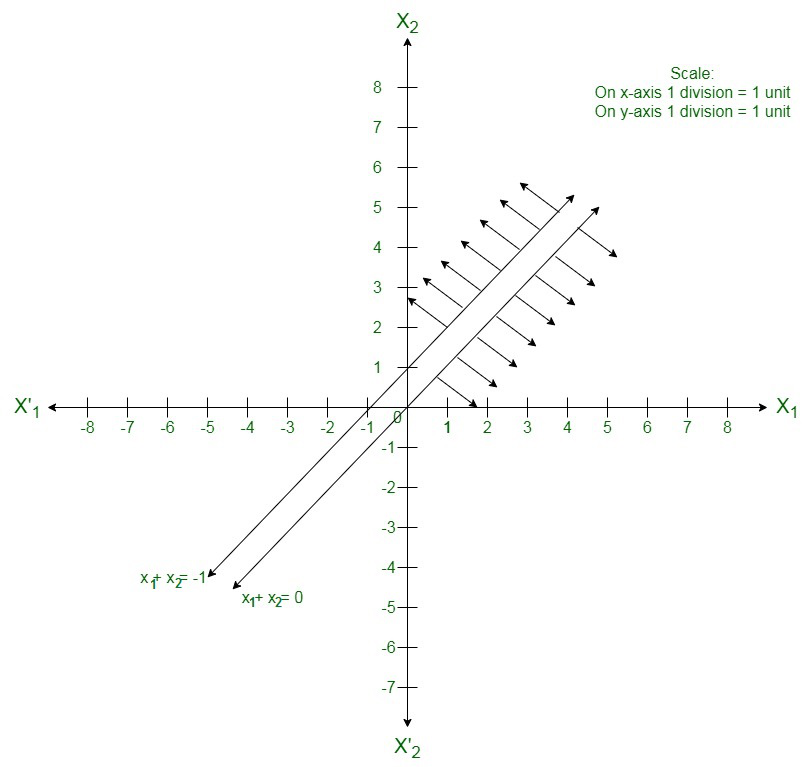Here we can see that the suitable area of the given LPP does not exist.

Whether you're preparing for your first job interview or aiming to upskill in this ever-evolving tech landscape, GeeksforGeeks Courses are your key to success. We provide top-quality content at affordable prices, all geared towards accelerating your growth in a time-bound manner. Join the millions we've already empowered, and we're here to do the same for you. Don't miss out - check it out now!

Related Tutorials